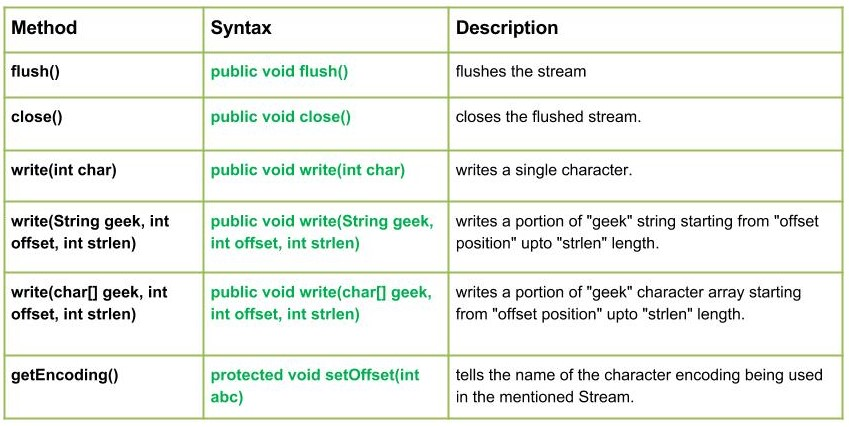Related Articles
Java.io.OutputStreamWriter Class methods
• Difficulty Level : Basic
• Last Updated : 06 Mar, 2017OutputStreamWriter class connects character streams to byte streams. It encodes Characters into bytes using a specified charset.
Declaration :

```public class OutputStreamWriter
extends Writer```

Constructors :

• OutputStreamWriter(OutputStream geek_out) : Creates a “geek_out” OutputStreamWriter that uses
a default charset for encoding.
• OutputStreamWriter(OutputStream geek_out, Charset geek_set) : Creates a “geek_out” OutputStreamWriterthat uses a “geek_ set” charset for encoding.
• OutputStreamWriter(OutputStream geek_out, CharsetEncoder encode) : Creates a “geek_out” OutputStreamWriter that uses a given encoder.
• OutputStreamWriter(OutputStream geek_out, String setName) : Creates a “geek_out” OutputStreamWriter that uses a named character set.

Methods:

• flush() : java.io.OutputStreamWriter.flush() flushes the stream.
Syntax :
```public void flush()
Parameters :
------
Return :
void
Exception :
-> IOException : if in case anu I/O error occurs.
```
• close() : java.io.OutputStreamWriter.close() closes the flushed stream.
Syntax :

```public void close()
Parameters :
------
Return :
void
Exception :
-> IOException : if in case anu I/O error occurs, e.g writing after closing the sream
```
• write(int char) : java.io.OutputStreamWriter.write(int char) writes a single character.
Syntax :
```public void write(int char)
Parameters :
char : character to be written
Return :
void
```
 `// Java code explaining the working of write(int char), flush(), close()`` ` `import` `java.io.*;``public` `class` `NewClass``{``    ``public` `static` `void` `main(String[] args)``    ``{        ``        ``try``        ``{``            ``// Creation of new OutputSreamWriter``            ``OutputStream g = ``new` `FileOutputStream(``"test.txt"``);``            ``OutputStreamWriter geeks_out1 = ``new` `OutputStreamWriter(g);`` ` `            ``// Creating an Input Stream``            ``FileInputStream in = ``new` `FileInputStream(``"test.txt"``);`` ` `            ``// Use of write(int char) :``            ``// Writing character values to the "test.txt"``            ``geeks_out1.write(``71``);``            ``geeks_out1.write(``69``);``            ``geeks_out1.write(``69``);``            ``geeks_out1.write(``75``);``            ``geeks_out1.write(``83``);`` ` `            ``// flush the stream``            ``geeks_out1.flush();`` ` `            ``// read what we write``            ``for` `(``int` `i = ``0``; i < ``5``; i++)``            ``{``                ``// Reading the content of "test.txt" file``                ``System.out.println(``"write(int char) : "` `+ (``char``) in.read());``            ``}``            ``geeks_out1.close();``        ``}``        ``catch` `(Exception ex)``        ``{``            ``System.out.println(``"Error"``);``            ``ex.printStackTrace();``        ``}``    ``}``}`

Output :

```write(int char) : G
write(int char) : E
write(int char) : E
write(int char) : K
write(int char) : S```
• write(String geek, int offset, int strlen) : java.io.OutputStreamWriter.write(String geek, int offset, int strlen) writes a portion of “geek” string starting from “offset position” upto “strlen” length.
Syntax :
```public void write(String geek, int offset, int strlen)
Parameters :
geek : string whose portion is to be written
offset : starting position from where to write
strlen : length upto which we need to write
Return :
void
Exception :
-> IOException : if in case any I/O error occurs.
```
 `// Java code explaining the working of write(String geek, int offset, int strlen))`` ` `import` `java.io.*;``public` `class` `NewClass``{``    ``public` `static` `void` `main(String[] args)``    ``{``        ``String geek = ``"GEEKSForGeeks"``;``        ``try``        ``{``            ``// Creation of new OutputSreamWriter``            ``OutputStream g = ``new` `FileOutputStream(``"test.txt"``);``            ``OutputStreamWriter geeks_out1 = ``new` `OutputStreamWriter(g);`` ` `            ``// Creating an Input Stream``            ``FileInputStream in = ``new` `FileInputStream(``"test.txt"``);`` ` `            ``// Use of writewrite(String geek, int offset, int strlen)) :``            ``// Writing character values to the "test.txt"``            ``geeks_out1.write(geek, ``4``, ``9``);`` ` `            ``// flush the stream``            ``geeks_out1.flush();`` ` `            ``// read what we write``            ``for` `(``int` `i = ``0``; i < ``5``; i++)``            ``{``                ``// Reading the content of "test.txt" file``                ``System.out.println(``"write(int char) : "` `+ (``char``) in.read());``            ``}``            ``geeks_out1.close();``        ``}``        ``catch` `(Exception ex)``        ``{``            ``System.out.println(``"Error"``);``            ``ex.printStackTrace();``        ``}``    ``}``}`

Output :

```write(int char) : S
write(int char) : F
write(int char) : o
write(int char) : r
write(int char) : G```
• write(char[] geek, int offset, int strlen) : java.io.OutputStreamWriter.write(char[] geek, int offset, int strlen) writes a portion of “geek” character array starting from “offset position” upto “strlen” length.
Syntax :
```public void write(char[] geek, int offset, int strlen)
Parameters :
geek : character array whose portion is to be written
offset : starting position from where to write
strlen : length upto which we need to write
Return :
void
Exception :
-> IOException : if in case anu I/O error occurs.
```
• getEncoding() : java.io.OutputStreamWriter.getEncoding() tells the name of the character encoding being used in the mentioned Stream.
If there is predefined name exists, then it is returned otherwise canonical name of the encoding is returned.
Returns Null, if the stream has been already closed.
Syntax :
```public String getEncoding()
Parameters :
------
Return :
Name of the charset encoding used
Exception :
-> IOException : if in case anu I/O error occurs.
```
 `// Java code explaining write(char[] geek, int offset, int strlen)``// and getEncoding() method`` ` `import` `java.io.*;``public` `class` `NewClass``{``    ``public` `static` `void` `main(String[] args)``    ``{``        ``char``[] geek = {``'G'``, ``'E'``, ``'E'``, ``'K'``, ``'S'``};``        ``try``        ``{``            ``// Creation of new OutputSreamWriter``            ``OutputStream g = ``new` `FileOutputStream(``"test.txt"``);``            ``OutputStreamWriter geeks_out1 = ``new` `OutputStreamWriter(g);`` ` `            ``// Creating an Input Stream``            ``FileInputStream in = ``new` `FileInputStream(``"test.txt"``);`` ` `            ``// Use of writewrite(char[] geek, int offset, int strlen)) :``            ``// Writing character values to the "test.txt"``            ``geeks_out1.write(geek, ``0``, ``3``);`` ` `            ``// flush the stream``            ``geeks_out1.flush();`` ` `            ``// read what we write``            ``for` `(``int` `i = ``0``; i < ``3``; i++)``            ``{``                ``// Reading the content of "test.txt" file``                ``System.out.println(``"char[] geek, int offset, int strlen) : "` `                                                            ``+ (``char``) in.read());``            ``}`` ` `            ``// get and print the encoding for this stream``            ``System.out.println(``"\nName of the charset : "``                                        ``+ geeks_out1.getEncoding());``             ` `            ``// Closing the OutputStreamWriter``            ``geeks_out1.close();``        ``}``         ` `        ``catch` `(Exception ex)``        ``{``            ``System.out.println(``"Error"``);``            ``ex.printStackTrace();``        ``}``    ``}``}`

Output :

```char[] geek, int offset, int strlen) : G
char[] geek, int offset, int strlen) : E
char[] geek, int offset, int strlen) : E

Name of the charset : UTF8```

This article is contributed by Mohit Gupta 🙂. If you like GeeksforGeeks and would like to contribute, you can also write an article using contribute.geeksforgeeks.org or mail your article to contribute@geeksforgeeks.org. See your article appearing on the GeeksforGeeks main page and help other Geeks.

Please write comments if you find anything incorrect, or you want to share more information about the topic discussed above.

Attention reader! Don’t stop learning now. Get hold of all the important Java Foundation and Collections concepts with the Fundamentals of Java and Java Collections Course at a student-friendly price and become industry ready. To complete your preparation from learning a language to DS Algo and many more,  please refer Complete Interview Preparation Course.

My Personal Notes arrow_drop_up